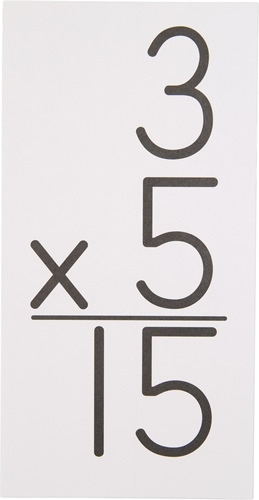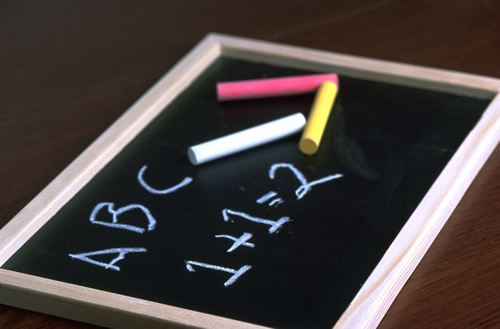•Math3rd - 4th grade Math :

Right now we are learning how to add single digit numbers, double digit numbers and even how to regroup when adding!  During class we use xtramath on the computers to help us practice our basic addition facts.

5th  - 6th grade Math :

This group of students are learning to multiply. We are using a program called Kickin - It Multiplication to help us learn our basic multiplication facts. Students have 1 minute to answer 50 multiplication questions. If they complete the task they move up a level. Students can click on the link at the bottom of the page to find practice activities.

Students are also using ipads to help them practice their math facts and skills.AdditionSubtractionMultiplication